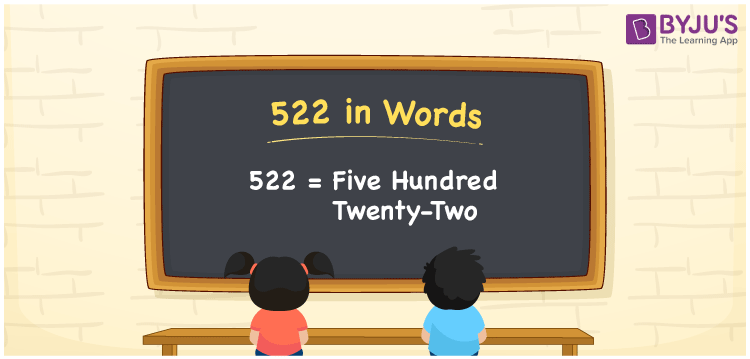# 522 in Words

522 in words is written as Five Hundred Twenty-two. The name of number 522 in English is “Five Hundred Twenty-two”. The word Five Hundred Twenty-two is used in many real-life situations. For example, there are Five Hundred Twenty-two students in a sports club. Thus, it is a counting number. Also, to represent the currency equal to 522, we can write it in words as Rupees Five Hundred Twenty-two or Five Hundred Twenty-two rupees. Therefore, it is necessary to learn the numbers in words, for the ease of understanding and expressing them.

 522 in words Five Hundred Twenty-two Five Hundred Twenty-two in Numbers 522

## 522 in English Words## How to Write 522 in Words?

If we know the place value of digits of 522, then we can easily express it in words. The place value is basically the position of a digit in a number. 522 is a three-digit number, therefore, we can specify the position of each digit of 522 in a place value chart. In the Indian numbering system, the order of place value of digits from right to left is given by:

 Hundreds Tens Ones 5 2 2

We can write it in expanded form as:

5 × Hundred + 2 × Ten + 2 × One

= 5 × 100 + 2 × 10 + 2 × 1

= 500 + 20 + 2

= 522

= Five Hundred Twenty-two

Therefore, 522 in words is written as Five Hundred Twenty-two.

522 is a natural number that precedes 521 and succeeds 523.

522 in words – Five Hundred Twenty-two

Is 522 an odd number? – No

Is 522 an even number? – Yes

Is 522 a perfect square number? – No

Is 522 a perfect cube number? – No

Is 522 a prime number? – No

Is 522 a composite number? – Yes

## Frequently Asked Questions on 522 in Words

Q1

### How to write 522 in words?

522 in English is written as “Five Hundred Twenty-two”.
Q2

### Is 522 an odd number or even number?

522 is an even number because it is wholly divisible by 2.
Q3

### Write Five Hundred Twenty-two in numbers.

Five Hundred Twenty-two in numbers is 522.